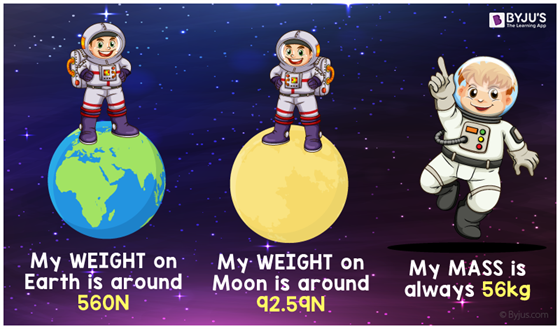Checkout JEE MAINS 2022 Question Paper Analysis : Checkout JEE MAINS 2022 Question Paper Analysis :

# Mass

## What is mass?

In Physics, mass is a physical property of a body. It is usually a measure of a body’s resistance to altering its state of motion when a force is exerted. It is measured by the strength of its mutual gravitational pull to other objects, its resistance to directional or accelerational changes, and the theory of relativity provides the mass-energy content of a system.

### Units of Mass

Kilogram (kg) is the SI unit (standard International System of Units) of mass. Where one kilogram is a 1000 grams (g), first described in 1795 as one cubic decimeter of water at the melting point of ice. The kilogram was reformulated as the mass of the international prototype of the kilogram in 1889, and as such is autonomous of the meter, or the properties of water. As of January 2013, there are several proposals for redefining the kilogram once more, including a proposal for describing it in terms of the Planck constant.

### Atomic Mass

Normally, the mass of objects are measured in relation to that of a kilogram, which is described as the mass of the international prototype kilogram (IPK), a platinum alloy cylinder put away in a safe environmentally monitored and fortified in a vault at the International Bureau of Weights and Measures (France). Yet, the IPK is not suitable for measuring the masses of atoms and particles of comparable scale, as it comprises of trillions of trillions of atoms, and has most definitely lost or gained a little mass over time in spite of the best efforts to avert this.

It is much simpler to compare an atom’s mass precisely to that of another atom. Thus, scientists formed the atomic mass unit (or Dalton). By definition, 1 u is exactly one-twelfth of the mass of a carbon-12 atom, and by when extended from that information a carbon-12 atom has a mass of exactly 12 u. This description, however, might be altered by the redefinition of SI base units, which will leave the value of 1 Dalton extremely close to one, but no longer precisely equal to it.

## Weight vs MassIn daily usage, mass and “weight” are frequently used interchangeably. For example, a person’s weight may be specified as 75 kg. In a constant gravitational field, an object’s weight is comparative to its mass, and thus it is unproblematic to make use of the same unit for both concepts. But due to slight differences in the strength of the Earth’s gravitational field at diverse places, the distinction becomes significant for measurements with an accuracy better than a few percent, and for locations far from the surface of the Earth, for instance in space or on other planets.

Weight and Mass are related by the equation,

W = m g

wherein W is the weight,

m is the mass of the body,

g is the gravitational acceleration, whose value is 9.8 m/s2 .

Theoretically, “mass” ( in kilograms) refers to an intrinsic property of a body. While “weight” (measured in newtons) measures an object’s resistance to differing from its natural course of free fall, which can be swayed by the nearby gravitational field. No matter how sturdy the gravitational field, objects in free fall are weightless, although they still have mass.